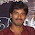### C# Convert List IEnumerable<T> to 2D multi-dimensional array

```//extension method
public static object[,] To2DArray<T>(this IEnumerable<T> lines, params Func<T, object>[] lambdas)
{
var array = new object[lines.Count(), lambdas.Count()];
var lineCounter = 0;
lines.ForEach(line =>
{
for (var i = 0; i < lambdas.Length; i++)
{
array[lineCounter, i] = lambdas[i](line);
}
lineCounter++;
});
return array;
}

[Test]
public void Test()
{
var lines = new List<Line>();
lines.Add(new Line() { Id=1, Name="One", Age=25 });
lines.Add(new Line() { Id = 2, Name = "Two", Age = 35 });
lines.Add(new Line() { Id = 3, Name = "Three", Age = 45 });

//Convert to 2d array
//[1,One,25]
//[2,Two,35]
//[3,Three,45]
var range = lines.To2DArray(x => x.Id, x => x.Name, x=> x.Age);

//test the result
for(var i=0;i<lines.Count;i++)
{
for(var j=0;j<3;j++)//3 lambdas passed to function
{
Console.Write(range[i,j]+",");
}
Console.WriteLine();
}
}

class Line
{
public int Id { get; set; }
public string Name { get; set; }
public int Age { get; set; }
}```

1.Hi, i'm getting error at lines.ForEach in To2DArray() method
error:
System.Collections.Generic.IEnumerable' does not contain a definition for 'Foreach' and no extension method 'Foreach' accepting a first argument of type 'System.Collections.Generic.IEnumerable' could be found (are you missing a using directive or an assembly reference?

2.I think I've used extension method for this

public static void ForEach(this IEnumerable enumeration, Action action)
{
foreach(T item in enumeration)
{
action(item);
}
}

3.This comment has been removed by the author.

4.This comment has been removed by the author.

5.< T > missing

6.Hello, this weekend is good for me, since this time i am reading this enormous informative article here at my home. convert money